## RD Sharma Class 10 Solutions Chapter 4 Quadratic Equations MCQS

These Solutions are part of RD Sharma Class 10 Solutions. Here we have given RD Sharma Class 10 Solutions Chapter 4 Quadratic Equations MCQS

Other Exercises

Mark the correct alternative in each of the following :
Question 1.
If the equation x² + 4x + k = 0 has real and distinct roots, then
(a) k < 4
(b) k > 4
(c) k ≥ 4
(d) k ≤ 4
Solution:
(a) In the equation x² + 4x + k = 0
a = 1, b = 4, c = k
D = b² – 4ac = (4)² – 4 x 1 x k = 16 – 4k
Roots are real and distinct
D > 0
=> 16 – 4k > 0
=> 16 > 4k
=> 4 > k
=> k < 4

Question 2.
If the equation x² – ax + 1 = 0 has two distinct roots, then
(a) |a| = 2
(b) |a| < 2
(c) |a| > 2
(d) None of these
Solution:
(c) In the equation x² – ax + 1 = 0
a = 1, b = – a, c = 1
D = b² – 4ac = (-a)² – 4 x 1 x 1 = a² – 4
Roots are distinct
D > 0
=> a² – 4 > 0
=> a² > 4
=> a² > (2)²
=> |a| > 2

Question 3.
If the equation 9x2 + 6kx + 4 = 0, has equal roots, then the roots are both equal to
(a) ± $$\frac { 2 }{ 3 }$$
(b) ± $$\frac { 3 }{ 2 }$$
(c) 0
(d) ± 3
Solution:
(a)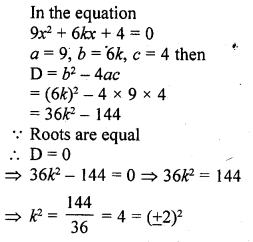Question 4.
If ax2 + bx + c = 0 has equal roots, then c =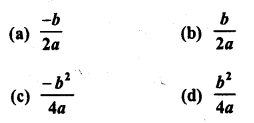Solution:
(d) In the equation ax2 + bx + c = 0
D = b2 – 4ac
Roots are equal
D = 0 => b2 – 4ac = 0
=> 4ac = b2
=> c = $$\frac { { b }^{ 2 } }{ 4a }$$

Question 5.
If the equation ax2 + 2x + a = 0 has two distinct roots, if
(a) a = ±1
(b) a = 0
(c) a = 0, 1
(d) a = -1, 0
Solution:
(a) In the equation ax2 + 2x + a = 0
D = b2 – 4ac = (2)2 – 4 x a x a = 4 – 4a2
Roots are real and equal
D = 0
=> 4 – 4a2 = 0
=> 4 = 4a2
=> 1 = a2
=> a2 = 1
=> a2 = (±1)2
=> a = ±1

Question 6.
The positive value of k for which the equation x2 + kx + 64 = 0 and x2 – 8x + k = 0 will both have real roots, is
(a) 4
(b) 8
(c) 12
(d) 16
Solution:
(d) In the equation x2 + kx + 64 = 0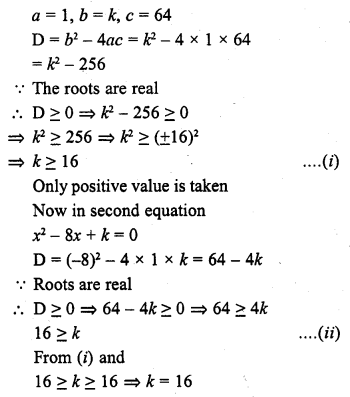Question 7.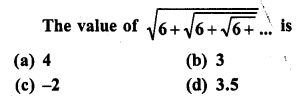Solution:
(b)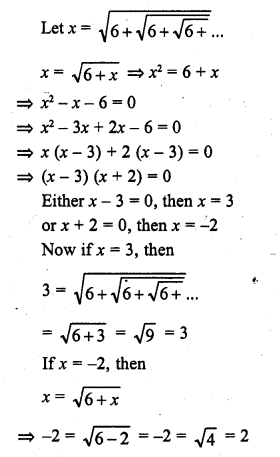Which is not possible
x = 3 is correct

Question 8.
If 2 is a root of the equation x2 + bx + 12 = 0 and the equation x2 + bx + q = 0 has equal roots, then q =
(a) 8
(b) – 8
(c) 16
(d) -16
Solution:
(c)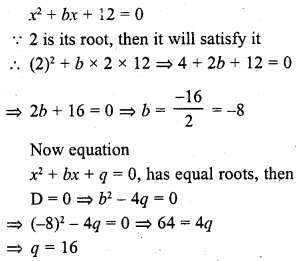Question 9.
If the equation (a2 + b2) x2 – 2 (ac + bd) x + c2 + d2 = 0 has equal roots, then
(a) ab = cd
(d) ab = √cd
Solution:
(b)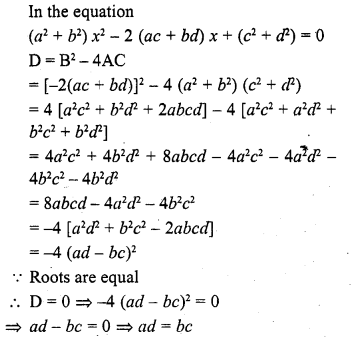Question 10.
If the roots of the equation (a2 + b2) x2 – 2b (a + c) x + (b2 + c2) = 0 are equal, then ;
(a) 2b = a + c
(b) b2 = ac
(c) b = $$\frac { 2ac }{ a + c }$$
(d) b = ac
Solution:
(b)Question 11.
If the equation x2 – bx + 1 = 0 does not possess real roots, then
(a) -3 < b < 3
(b) -2 < b < 2
(c) b > 2
(d) b < -2
Solution:
(b)Question 12.
If x = 1 is a common root of the equations ax2 + ax + 3 = 0 and x2 + x + b = 0, then ab =
(a) 3
(b) 3.5
(c) 6
(d) -3
Solution:
(a) In the equation
ax2 + ax + 3 = 0 and x2 + x + b = 0
Substituting the value of x = 1, then in ax2 + ax + 3 = 0Question 13.
If p and q are the roots of the equation x2 – px + q + 0, then
(a) p = 1, q = -2
(b) p = 0, q = 1
(c) p = -2, q = 0
(d) p = -2, q = 1
Solution:
(a)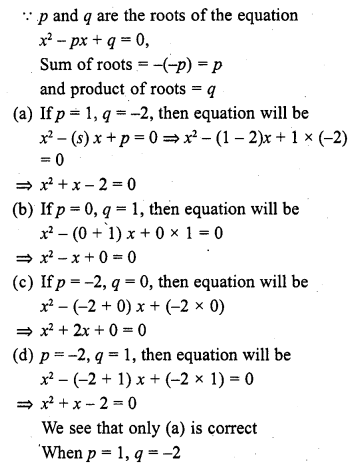Question 14.
If a and b can take values 1, 2, 3, 4. Then the number of the equations of the form ax2 + bx + 1 = 0 having real roots is
(a) 10
(b) 7
(c) 6
(d) 12
Solution:
(b)
ax2 + bx + 1 = 0
D = b2 – 4a = b2 – 4a
Roots are real
D ≥ 0
=> b2 – 4a ≥ 0
=> b2 ≥ 4a
Here value of b can be 2, 3 or 4
If b = 2, then a can be 1,
If b = 3, then a can be 1, 2
If b = 4, then a can be 1, 2, 3, 4
No. of equation can be 7

Question 15.
The number of quadratic equations having real roots and which do not change by squaring their roots is
(a) 4
(b) 3
(c) 2
(d) 1
Solution:
(c) There can be two such quad, equations whose roots can be 1 and 0
The square of 1 and 0 remains same
No. of quad equation are 2

Question 16.
If (a2 + b2) x2 + 2(ab + bd) x + c2 + d2 = 0 has no real roots, then
(b) ab = cd
(c) ac = bd
Solution:
(d)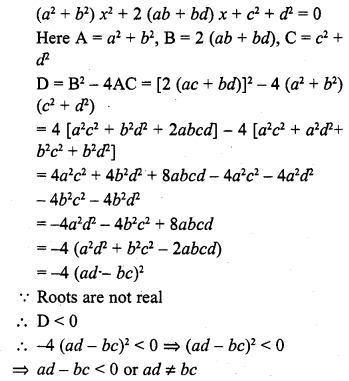Question 17.
If the sum of the roots of the equation x2 – x = λ (2x – 1) is zero, then λ =
(a) -2
(b) 2
(c) – $$\frac { 1 }{ 2 }$$
(d) $$\frac { 1 }{ 2 }$$
Solution:
(c)Question 18.
If x = 1 is a common root of ax2 + ax + 2 = 0 and x2 + x + b = 0 then, ab =
(a) 1
(b) 2
(c) 4
(d) 3
Solution:
(b)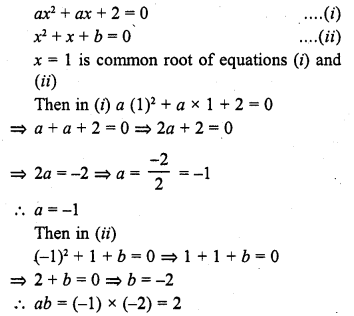Question 19.
The value of c for which the equation ax2 + 2bx + c = 0 has equal roots is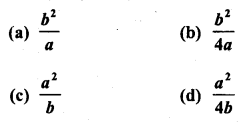Solution:
(a)Question 20.
If x2 + k (4x + k – 1) + 2 = 0 has equal roots, then k =Solution:
(b)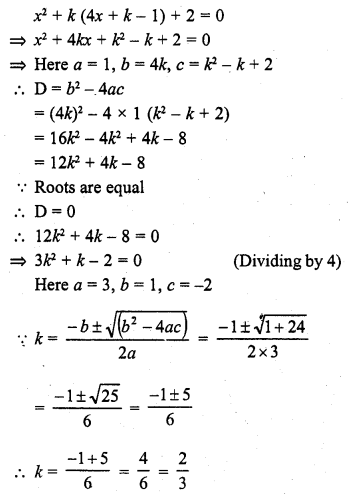Question 21.
If the sum and product of the roots of the equation kx2 + 6x + 4k = 0 are equal, then k =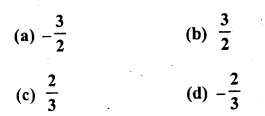Solution:
(b)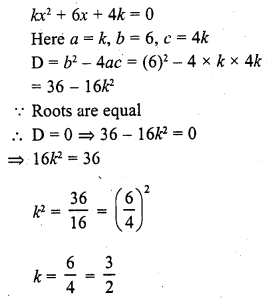Question 22.
If sin α and cos α are the roots of the equations ax2 + bx + c = 0, then b2 =
(a) a2 – 2ac
(b) a2 + 2ac
(b) a2 – ac
(d) a2 + ac
Solution:
(b)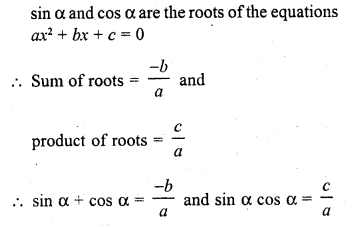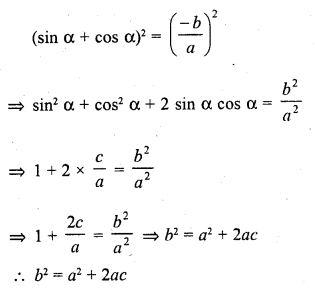Question 23.
If 2 is a root of the equation x2 + ax + 12 = 0 and the quadratic equation x2 + ax + q = 0 has equal roots, then q =
(a) 12
(b) 8
(c) 20
(d) 16
Solution:
(d)Question 24.
If the sum of the roots of the equation x2 – (k + 6) x + 2 (2k – 1) = 0 is equal to half of their product, then k =
(a) 6
(b) 7
(c) 1
(d) 5
Solution:
x2 – (k + 6) x + 2 (2k – 1) = 0
Here a = 1, b = – (k + 6), c = 2 (2k – 1)Question 25.
If a and b are roots of the equation x2 + ax + b = 0, then a + b =
(a) 1
(b) 2
(c) -2
(d) -1
Solution:
(d) a and b are the roots of the equation x2 + ax + b = 0
Sum of roots = – a and product of roots = b
Now a + b = – a
and ab = b => a = 1 ….(i)
2a + b = 0
=> 2 x 1 + b = 0
=> b = -2
Now a + b = 1 – 2 = -1

Question 26.
A quadratic equation whose one root is 2 and the sum of whose roots is zero, is
(a) x2 + 4 = 0
(b) x2 – 4 = 0
(c) 4x2 – 1 = 0
(d) x2 – 2 = 0
Solution:
(b) Sum of roots of a quad, equation = 0
One root = 2
Second root = 0 – 2 = – 2
and product of roots = 2 x (-2) = – 4
Equation will be
x2 + (sum of roots) x + product of roots = 0
x2 + 0x + (-4) = 0
=> x2 – 4 = 0

Question 27.
If one root of the equation ax2 + bx + c = 0 is three times the other, then b2 : ac =
(a) 3 : 1
(b) 3 : 16
(c) 16 : 3
(d) 16 : 1
Solution:
(c)Question 28.
If one root of the equation 2x2 + kx + 4 = 0 is 2, then the other root is
(a) 6
(b) -6
(c) -1
(d) 1
Solution:
(d) The given quadratic equation 2x2 + kx + 4 = 0
One root is 2
Product of roots = $$\frac { c }{ a }$$ = $$\frac { 4 }{ 2 }$$ = 2
Second root = $$\frac { 2 }{ 2 }$$ = 1

Question 29.
If one root of the equation x2 + ax + 3 = 0 is 1, then its other root is
(a) 3
(b) -3
(c) 2
(d) -2
Solution:
(a) The quad, equation is x2 + ax + 3 = 0
One root =1
and product of roots = $$\frac { c }{ a }$$ = $$\frac { 3 }{ 1 }$$ = 3
Second root = $$\frac { 3 }{ 1 }$$ = 3

Question 30.
If one root of the equation 4x2 – 2x + (λ – 4) = 0 be the reciprocal of the other, then λ =
(a) 8
(b) -8
(c) 4
(d) -4
Solution:
(a)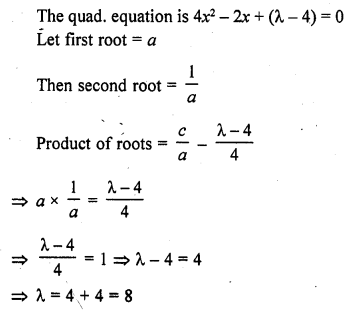Question 31.
If y = 1 is a common root of the equations ay2 + ay + 3 = 0 and y2 + y + b = 0, then ab equals
(a) 3
(b) – $$\frac { 1 }{ 2 }$$
(c) 6
(d) -3 [CBSE 2012]
Solution:
(a)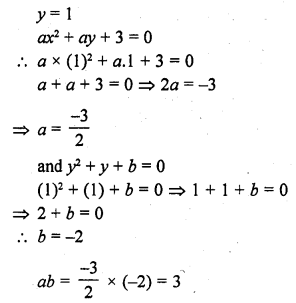Question 32.
The values of k for which the quadratic equation 16x2 + 4kx + 9 = 0 has real and equal roots are
(a) 6, – $$\frac { 1 }{ 6 }$$
(b) 36, -36
(c) 6, -6
(d) $$\frac { 3 }{ 4 }$$ , – $$\frac { 3 }{ 4 }$$ [CBSE 2014]
Solution:
(c) 16x2 + 4kx + 9 = 0
Here a = 16, b = 4k, c = 9
Now D = b2 – 4ac = (4k)2 – 4 x 16 x 9 = 16k2 – 576
Roots are real and equal
D = 0 or b2 – 4ac = 0
=> 16k2 – 576 = 0
=> k2 – 36 = 0
=> k2 = 36 = (± 6)2
k = ± 6
k = 6, -6

Hope given RD Sharma Class 10 Solutions Chapter 4 Quadratic Equations MCQS are helpful to complete your math homework.

If you have any doubts, please comment below. Learn Insta try to provide online math tutoring for you.